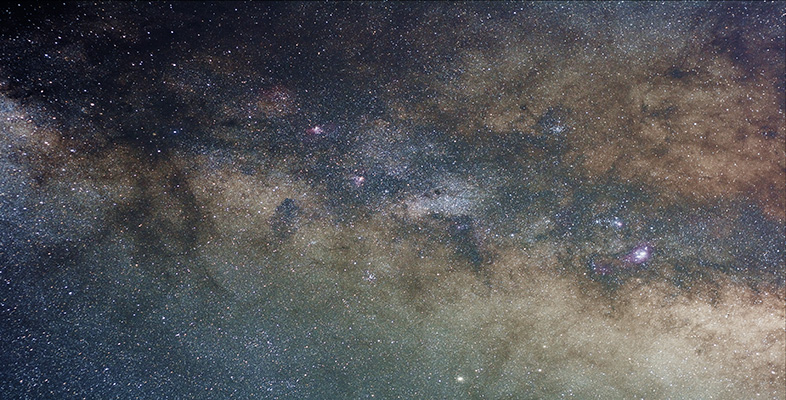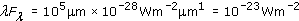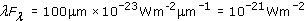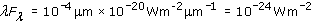Introduction to active galaxies

Start this free course now. Just create an account and sign in. Enrol and complete the course for a free statement of participation or digital badge if available.

Free course

# 7.6 Questions

## Question 15

Suppose that a galaxy has emission lines in its optical spectrum. A line of wavelength 654.3 nm is broadened by 2.0 nm. Estimate the velocity dispersion of the gas giving rise to the broadened spectral line. Is it likely to be a normal galaxy?

For the gas motion use Equation 3.1, Δλ/λ = Δv/c, where Δv is the velocity dispersion. Then Δλ/λ = 2.0 nm/654.3 nm ≈ 0.0030.

Thus the overall spread of internal speeds is Δv ≈ 0.0030 × c ≈ 900 km s−1, which is too large for a normal galaxy.

## Question 16

Calculate λFλ flux densities in W m−2 in the radio, the far infrared and the X-ray regions, given the Fλ and λ values listed in Table 2. Which wavelength region dominates?

### Table 2

RegionλFλ/W m−2 μm−1λFλ/W m−2
far-IR100 μm10−23
X-ray10−10 m10−20

In the radio wave region, λ = 105 μm soIn the far infrared region λ = 100 μm so thatIn the X-ray region, λ = 10−4 μm soThe largest of these λFλ values is 10−21 W m−2, so we conclude that the far-infrared emission dominates.

## Question 17

Suppose that an unusual galaxy has broadband spectral flux densities Fλ at wavelengths 500 nm, 5 μm and 50 μm, of 10−27, 10−28, and 10−28 W m−2 μm−1, respectively. By calculating λFλ, comment on whether it is likely to be a normal or an active galaxy.

The wavelengths λ are 0.5 μm, 5 μm and 50 μm, therefore the λFλ values are 5 × 10−28 W m−2, 5 × 10−28 W m−2 and 5 × 10−27 W m−2, respectively. The largest of these values is 5 × 10−27 W m−2, so the dominant flux is at 50 μm, which is in the far infrared. The object is likely to be either a starburst galaxy or an active galaxy.

## Question 18

A particular galaxy has a large luminosity at X-ray wavelengths. One astronomer believes it to be a galaxy that happens to contain a large number of separate X-ray stars. Another astronomer believes that the X-rays indicate an active galaxy. How, by measuring the spectrum of the galaxy, could this question be resolved?

If the galaxy were active, one would expect to see strong emission lines in the optical and spectral excesses at non-optical wavelengths.